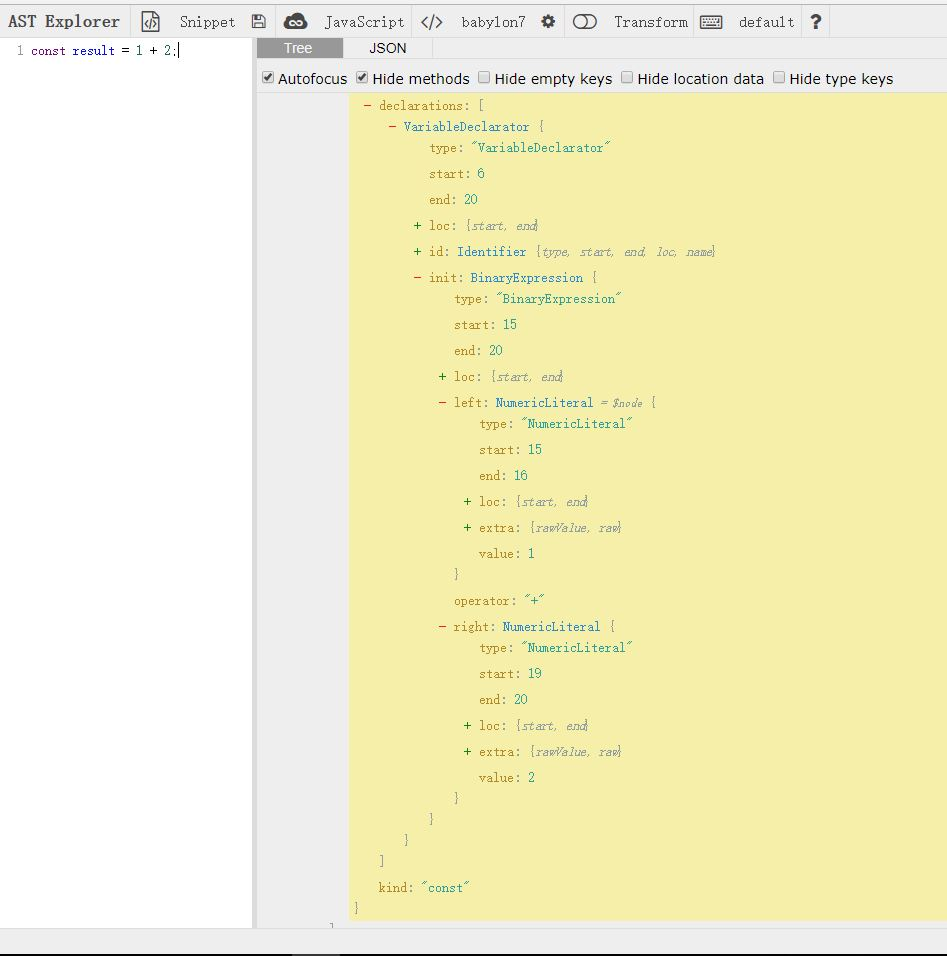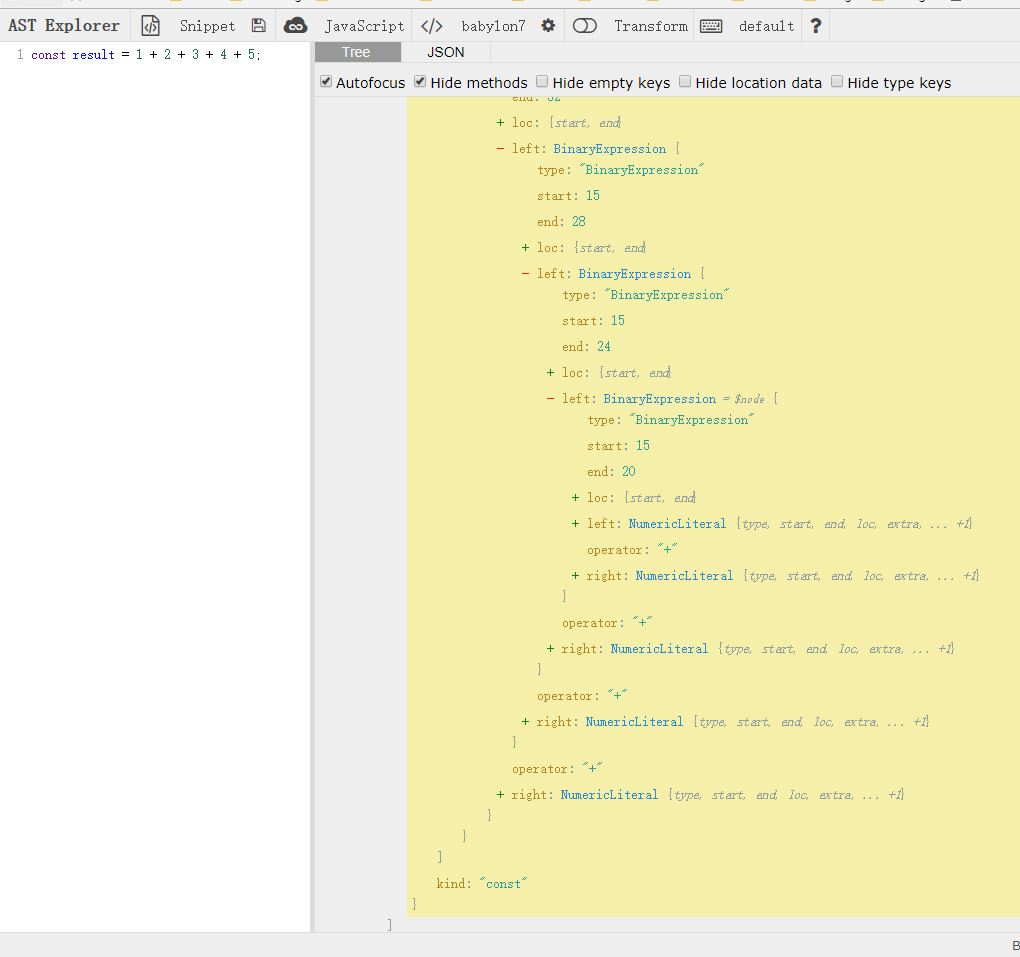1. 你了解过Babel吗？

1. 写过Babel插件吗

1. 如果让你写一个插件，你能写的出来吗?

### 预览

Before:

`const result = 1 + 2 + 3 + 4 + 5;`

After:

`const result = 15;`

```setTimeout(function(){
// do something
}, 1000 * 2) // 插件要做的事，就是把 1000 * 2 替换成 2000```

### 开工

```module.export = function(babel){
return {
visitor:{
}
}
}```

visitor是对各类型的AST节点做处理的地方，那么我们怎么知道Babel生成了的AST有哪些节点呢？```var babel = require('babel-core');
var t = require('babel-types');

const visitor = {
BinaryExpression(path) {
const node = path.node;
let result;
// 判断表达式两边，是否都是数字
if (t.isNumericLiteral(node.left) && t.isNumericLiteral(node.right)) {
// 根据不同的操作符作运算
switch (node.operator) {
case "+":
result = node.left.value + node.right.value;
break
case "-":
result = node.left.value - node.right.value;
break;
case "*":
result =  node.left.value * node.right.value;
break;
case "/":
result =  node.left.value / node.right.value;
break;
case "**":
let i = node.right.value;
while (--i) {
result = result || node.left.value;
result =  result * node.left.value;
}
break;
default:
}
}

// 如果上面的运算有结果的话
if (result !== undefined) {
// 把表达式节点替换成number字面量
path.replaceWith(t.numericLiteral(result));
}
}
};

module.exports = function (babel) {
return {
visitor
};
}```

```const babel = require("babel-core");

const result = babel.transform("const result = 1 + 2;",{
plugins:[
require("./index")
]
});

console.log(result.code); // const result = 3;`````````表达式( 表达式( 表达式( 表达式(1 + 2) + 3) + 4) + 5)
``````

```    // 判断表达式两边，是否都是数字
if (t.isNumericLiteral(node.left) && t.isNumericLiteral(node.right)) {}```

``````表达式( 表达式( 表达式(3+ 3) + 4) + 5)
``````

``````表达式( 表达式(6 + 4) + 5)

15
``````
```    // 如果上面的运算有结果的话
if (result !== undefined) {
// 把表达式节点替换成number字面量
path.replaceWith(t.numericLiteral(result));

let parentPath = path.parentPath;

// 向上遍历父级节点
parentPath && visitor.BinaryExpression.call(this, parentPath);
}```

`const result = 100 + 10 - 50` >>> `const result = 60;`

`const result = (100 / 2) + 50` >>> `const result = 100;`

`const result = (((100 / 2) + 50 * 2) / 50) ** 2` >>> `const result = 9;`

...

...